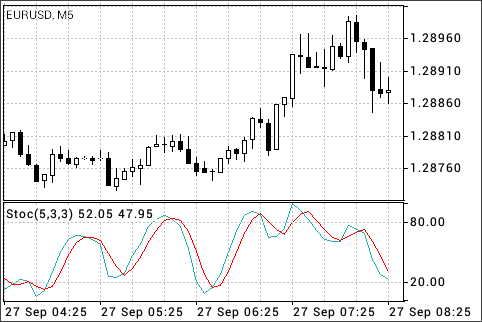MetaTrader 5 Android 帮助图表指标振荡器 (Oscillators)随机振荡器 (Stochastic Oscillator)

# 随机振荡器 (Stochastic Oscillator)

• 当振荡器 (或 %K 或 %D) 降至特定等级以下 (例如，20) 然后又回升到该等价以上，可以买入。当振荡器上到特定等级以上 (例如，80) 然后又回落到该等级以下，可以卖出。
• 当 %K 线上涨高过 %D 则买入。当 %K 线下跌低于 %D 则卖出。
• 寻找背离。比如：价格创出一系列新高，而随机振荡器未能突破其前高。## 计算

• %K 周期。这是用于计算随机振荡器的时间周期数。
• %K 慢速周期。该数值控制着 %K 的内部平滑。数值 1 被认为是快速随机; 数值 3 被认为是慢速的。
• %D 周期。这是计算 %K 的移动平均线时使用的时间周期数。
• %D 方法。用于计算 %D 的方法 (例如，指数、简单、平滑、或者加权)。

%K 公式：

%K = (CLOSE - MIN (LOW (%K))) / (MAX (HIGH (%K)) - MIN (LOW (%K))) * 100

CLOSE ― 本期收盘价;
MIN (LOW (%K)) ― %K 周期最低价的最小值;
MAX (HIGH (%K)) ― 周期最高价的最大值。

%D 移动平均线根据以下公式计算:

%D = SMA (%K, N)

N ― 平滑周期;
SMA ― 简单移动平均线